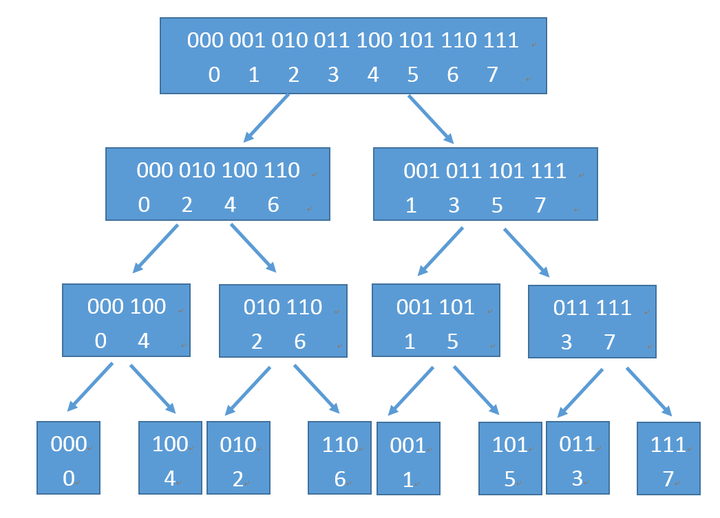# arrow_backfft共7篇文章zcmimi
2020-08-27 22:46:00zcmimi
2020-02-25

## FFT

fst fst tle

DFT: 离散傅里叶变换

IDFT: 离散傅里叶逆变换

FFT: 快速傅里叶变换

FNTT/NTT: 快速傅里叶变换的优化版

FWT: 快速沃尔什变换,利用类似FFT的东西解决一类卷积问题

MTT: 毛爷爷的FFT,非常nb/任意模数

FMT: 快速莫比乌斯变化

len_a=n,len_b=mzc
2020-07-25 23:00:00

\displaystyle \begin{aligned} P(x) &=\sum_{i=0}^{m-1}[A(i)-B(x-m+i+1)]^2A(i)B(x-m+i+1)\\ &=\sum_{i=0}^{m-1}[S(m-i+1)-B(x-m+i+1)]^2S(m-i+1)B(x-m+i+1)\\ &=\sum_{i=0}^{m-1}S(m-i+1)^3B(x-m+i+1)+\\ &\quad\sum_{i=0}^{m-1}B(x-m+i+1)^3S(m-i+1)-\\ &\quad2\sum_{i=0}^{m-1}S(m-i+1)^2B(x-m+i+1)^2 \end{aligned}

\displaystyle P(x)=\sum_{i+j=x}S(i)^3B(j)+S(i)B(j)^3-2S(i)^2B(j)^2zc
2020-07-05 13:10:00

A_i=[S_i=a],B_i=[S_i=b]zc
2020-03-08 15:07:00zc
2020-02-08 14:58:00

 E_i=\frac{F_i}{q_i}=\sum_{j=1}^{i-1}\frac{q_j}{(i-j)^2}-\sum_{j=i+1}^n \frac{q_j}{(j-i)^2} 

f_i=q_i,g_i=\frac 1{i^2},f_0=0,g_0=0

 E_i=\sum_{j=1}^i f_j\cdot g_{i-j}-\sum_{j=i}^n f_j\cdot g_{j-i} 

 \sum_{j=i}^n f_j\cdot g_{j-i} \\ =\sum_{j=0}^{n-i} f_{i+j}\cdot g_j 

t=n-i,f'_i=f_{n-i}

 =\sum_{j=i}^t g_j\cdot f'_{t-i}zc
2020-02-05 12:21:00

## FFT

fst fst tle

DFT: 离散傅里叶变换

IDFT: 离散傅里叶逆变换

FFT: 快速傅里叶变换

FNTT/NTT: 快速傅里叶变换的优化版

FWT: 快速沃尔什变换,利用类似FFT的东西解决一类卷积问题

MTT: 毛爷爷的FFT,非常nb/任意模数

FMT: 快速莫比乌斯变化

len_a=n,len_b=m

## 多项式

### 系数表示法

f(10)=6+5\cdot10+4\cdot10^2+3\cdot10^3+2\cdot 10^4+1\cdot 10^5

### 点值表示法

n个点x_1,x_2, \dots ,x_n

f(x)(x_1,y_1),(x_2,y_2),\dots (x_n,y_n)唯一确定

C(x)=A(x)-B(x),那么C(x)也是一个n-1次多项式,且\forall i\in[1,n], C(x_i)=0

## 复数

i是虚树单位,i^2=-1,i=\sqrt{-1}

### 复数运算法则:

(a+bi)+(c+di)=(a+c)+(b+d)i

(a+bi)-(c+di)=(a-c)+(b-d)i

(a+bi)\cdot(c+di)=(ac-bd)+(ad+bc)i

### 共轭复数

z\cdot z' = a^2 + b^2

|z|=|z'|(模长相等,到原点的距离相等)

## 单位根

\omega^n=1\omegan次单位根

\omega_n^0=\omega_n^n=1,在复平面上对应的点为(1,0)

\omega_n^k=e^{i 2\pi \frac kn} (可以理解为从\omega_n^0(1,0)开始走长度为2\pi \frac kn的圆弧后到达的位置)

https://www.bilibili.com/video/av63666593

https://www.bilibili.com/video/av79134103

\omega_n^k= \cos 2\pi\frac kn+ i \sin 2\pi \frac kn

\omega_{2n}^{2k}=\omega_n^k

\omega_{2n}^{2k}=\cos 2\pi\frac {2k}{2n}+ i \sin 2\pi \frac {2k}{2n}=\cos 2\pi\frac kn+ i \sin 2\pi \frac kn=\omega_n^k

\omega_n^0=\omega_n^n=1

\omega_n^{k+\frac n2}=-\omega_n^k

\omega _{n}^{\frac{n}{2}}=\cos\frac{n}{2}\cdot\frac{2\pi}{n}+i\sin\frac{n}{2}\cdot\frac{2\pi}{n}=-1 (也就是上面说的e^{i\pi})

## DFT

A(x)=(a_0+a_2x^2+a_4x^4+\dots+a_{n-2}x^{n-2})+x(a_1+a_3x^2+a_5x^4+...+a_{n-1}x^{n-1})

A_1(x)=a_0+a_2x+a_4x^2+\dots+a_{n-2}x^\frac{n-2}2,A_2(x)=a_1+a_3x+a_5x^2+...+a_{n-1}x^\frac{n-2}2

0 \le k \le \frac{n}{2}-1,k\in Z

A(\omega_n^k)=A_1(\omega_\frac n2 ^k)+\omega_n^k\cdot A_2(\omega_\frac n2 ^k)

A(\omega_n^{k+\frac n2})=A_1(\omega_n^{2k+n})+\omega_n^{k+\frac n2}\cdot A_2(\omega_n^{2k+n})

\because \omega_n^{k+\frac n2}=-\omega_n^k,\omega_n^{2k+n}=\omega_\frac n2^k

\therefore A(\omega_n^{k+\frac n2})=A_1(\omega_\frac n2^k)-\omega_n^k\cdot A_2(\omega_\frac n2^k)

## IDFT

y_0,y_1,y_2,...,y_{n-1}是多项式A(x)变换后的点值

(d_0,d_1,\dots,d_{n-1})(a_0,a_1,\dots,a_{n-1})离散傅里叶变换后的结果

c_kF(x)x=\omega_n^{-k}时的点值表示

$$ck=\sum{i=0}^{n-1}d_i\cdot(\omegan^{-k})^i \ =\sum{i=0}^{n-1}[\sum_{j=0}^{n-1}a_j(\omega_n^i)^j]\cdot(\omegan^{-k})^i \ =\sum{j=0}^{n-1}aj\sum{i=0}^{n-1}(\omega_n^i)^j\cdot(\omegan^{-k})^i \ =\sum{j=0}^{n-1}aj\sum{i=0}^{n-1}(\omega_n^i)^{j-k}$$

j=k\sum_{i=0}^{n-1}(\omega_n^i)^{j-k}=\sum_{i=0}^{n-1}1=n

j\not = k

\sum_{i=0}^{n-1}(\omega_n^i)^{j-k}=

\sum_{i=0}^{\frac{n-1}2}(\omega_n^i)^{j-k}+(\omega_n^{i+\frac{n-1}2})^{j-k}=0

\therefore \sum_{i=0}^{n-1}(\omega_n^i)^{j-k}=[j=k]\cdot n

\therefore c_k=\sum_{j=0}^{n-1}a_j\sum_{i=0}^{n-1}(\omega_n^i)^{j-k}=\sum_{j=0}^{n-1}a_j [j=k]\cdot n=na_k

\therefore a_k=\frac{c_k}n

1. (\omega_n^0,\omega_n^{-1},\omega_n^{-2},\dots,w_n^{-(n-1)})作为差值节点,将点值再做一次DFT

得到(c_0,c_1,c_2,\dots,c_{n-1})

2. (\frac{c_0}n,\frac{c_1}n,\frac{c_2}n,\dots,\frac{c_{n-1}}n)就是原来的(a_0,a_1,a_2,\dots,a_{n-1})

## 实现

LG 3803 【模板】多项式乘法（FFT）

FstFstTLE

### 迭代实现#### 蝴蝶操作

A_1(\omega_{\frac n2}^k)A_2(\omega_{\frac n2}^k)分别存放在a_ka_{k+\frac n2}

A_1(\omega_n^k)A_2(\omega_n^k)要存放在buf(k)buf(k+\frac n2)

buf(k+\frac n2)= a(k) - t

buf(k) = a(k)+t

Search
search